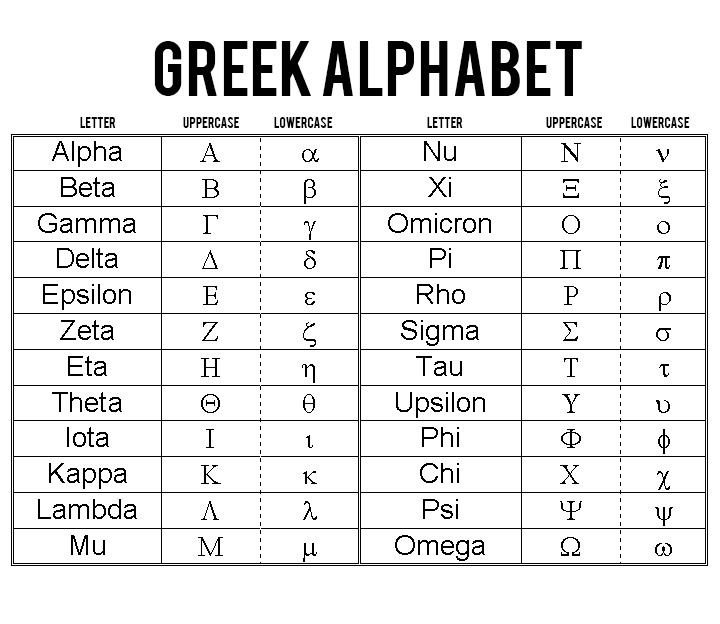# A Master List of All the Greek Letters as Used in Engineering

## Letters in the Greek alphabet are used to denote constants, functions, and variables.

Share via

Even in the first mathematics subjects in engineering like algebra and trigonometry, letters in the Greek alphabet are used to denote constants, functions, and variables representing quantities in concepts, figures, and formulas. These letters are carried on to advanced subjects representing different notations, both in capital letter and small letters.

But one may ask: why wouldn’t math and science scholars use the English alphabet instead? Why the need for the Greek alphabet?

There are plenty of representations required in the study of math and science that using the English alphabet alone will not be enough. It was necessary for another set of symbols because scholars ran out of letters.

Using Greek letters was called for since most academics in the Western world were trained in classical Greek at school. It was a candidate that wasn’t opposed. Plus, much of the basic math and science concepts were discovered by scientists and mathematicians of Greek descent.

Since then, the Greek letters, along with other alphabets like Roman, became a convention now used in engineering.

But unlike the English alphabet, the Greek alphabet is only composed of 24 letters. It was first used in 800 BCE with the Phoenician alphabet as its parent. As studies in different areas of knowledge developed, they were used comprehensively only to have various descriptions across topics.

Below is the list of the Greek alphabet with its corresponding but not exclusive uses in engineering:Alpha is used as absorption factor, angles, angular acceleration, attenuation constant, common-base currentam pilifition factor, deviation of state parameter, temperature coefficient of resistance, thermal expansion coefficient, and thermal diffusivity.

Read more  HVAC Security Online Threats and How To Mitigate

Beta is used as angles, common-emitter current-amplification factor, flux density, phase constant, and wavelength constant.

Gamma is used as electrical conductivity and Grueneisen parameter.

Delta is used as angles, damping coefficient (decay constant), decrement, increment, and secondary-emission ratio.

Epsilon is used as capacitivity, dielectric current, electric field intensity, electron energy, emissivity, permittivity, and constant of 2.7128 or base of natural logarithms.

Zeta is used as coefficients, coordinates and impedance.

Eta is used as chemical potential, dielectric susceptibility, efficiency, hysteresis, intrinsic impedance of a medium, and intrinsic standoff ratio.

Theta is used as angle of rotation, angles, angular phase displacement, reluctance, thermal resistance, and transit angle.

Iota is used as inertia.

Kappa is used as coupling coefficient and susceptibility.

Lambda is used as line density of charge, permanence, photosensitivity, and wavelength.

Mu is used as amplification factor, magnetic permeability, micron, mobility, permeability and prefix micro.

Nu is used as reflectivity.

Xi is used as output coefficient.

Omicron is not at all used in math and science, but part of Greek alphabet.

Pi is used as Peltier coefficient and ratio of circumference to its diameter valued at 3.1416.

Rho is used as reflection coefficient, reflection factor, resistivity, and surface density of charge.

Sigma is used as conductivity, Stefan-Boltzmann constant, summation, and surface density of charge.

Tau is used as period, propagation constant, Thomson coefficient, time constant, time-phase displacement, and transmission factor.

Phi is used as angles, coefficient of performance, contact potential, magnetic flux, phase angle, phase displacement, and radiant flux.

Read more  This Modified Minecraft Game Can Teach You Chemistry

Chi is used as angles.

Psi is used as angles, dielectric flux, displacement flux, and phase difference.

Omega is used as angular frequency, angular velocity, Ohms, resistance, and solid angle.

Share via